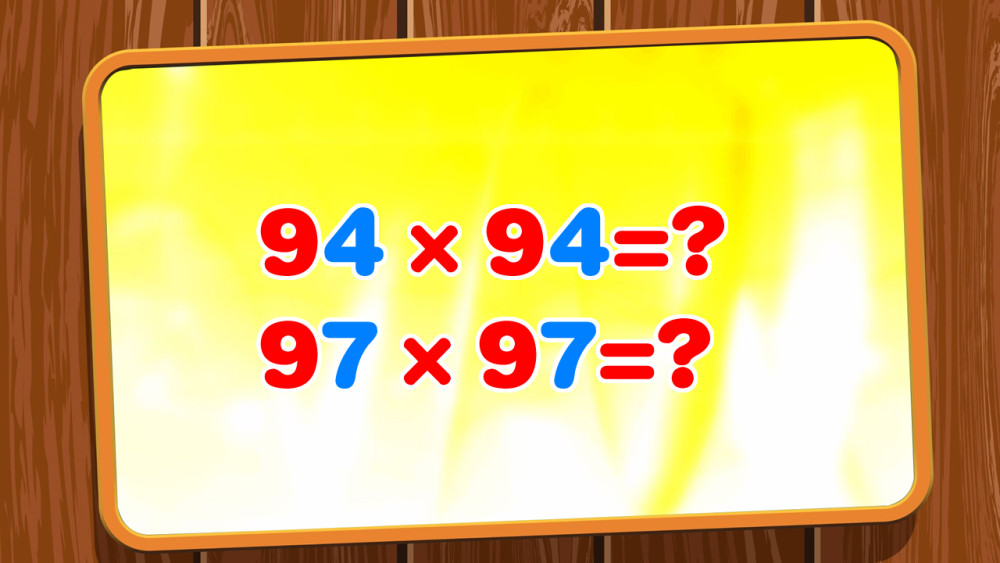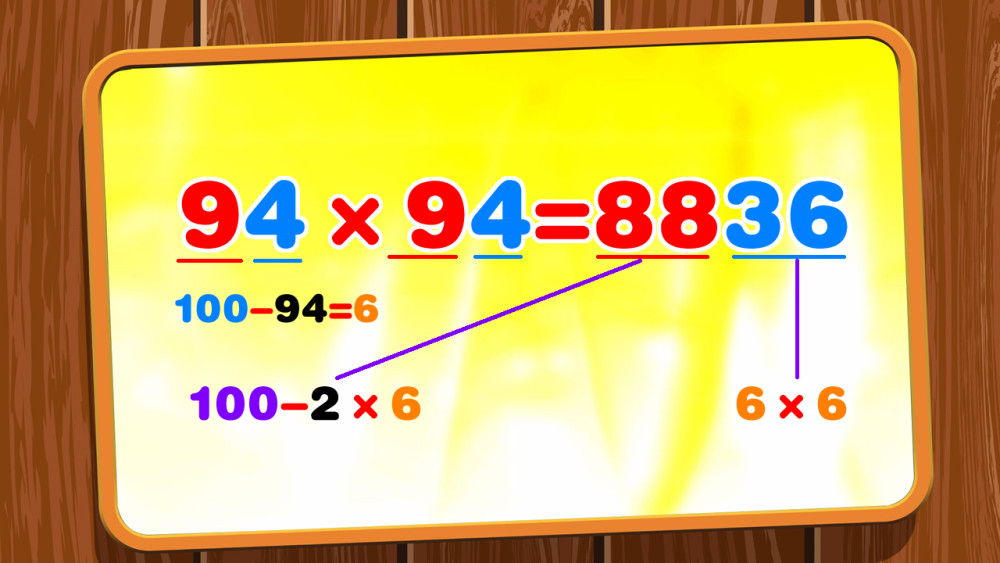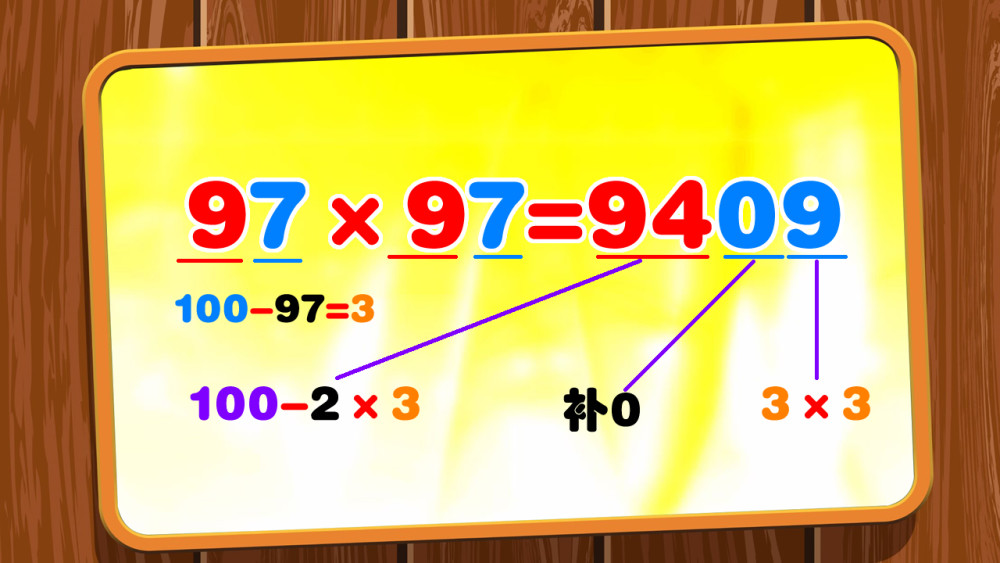# 九十几的自乘积，即九十几的平方，你能很快计算出来吗？

94×94=?

97×97=?94×94

=(100-6)×(100-6)

=(100-2×6)×100＋6×6

=8800＋36

=8836

97×97

=(100-3)×(100-3)

=(100-2×3)×100＋3×3

=9400＋9

=9409(100-a)^2

=(100-a)(100-a)

=(100-a)×100-(100-a)×a

=(100-a)×100-100a+a^2

=(100-a-a)×100+a^2

=(100-2a)×100+a^2